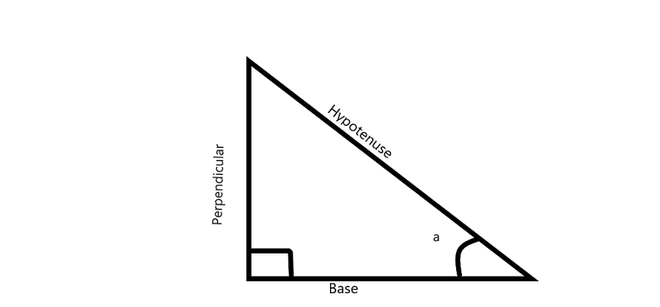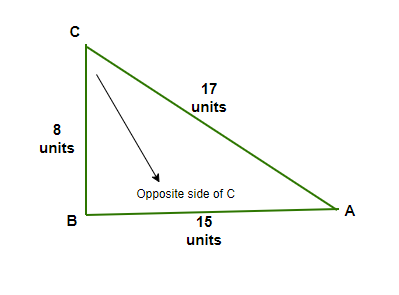Related Articles

# Consider a right triangle ABC, right angled at B and If AC = 17 units and BC = 8 units, then determine all the trigonometric ratios of angle C.

• Last Updated : 03 Sep, 2021

Trigonometry is a branch of mathematics that gives the relationships between side lengths and angles of triangles. there are six angles and their function for calculation in trigonometry. All trigonometric angles have fixed values and they can also be defined by the ratio of the lengths of the sides in a right-angled triangle. For instance, sin30° is equal to 1/2 and cos 30° is equal to √3/2, cos∅ is also defined as the ratio of base to its hypotenuse in a right-angled triangle, and so on. The six trigonometric ratios are⇢ sine (sin), cosine (cos), tangent (tan), cotangent (cot), cosecant (cosec), and, secant (sec).

### Trigonometry Ratios

The six trigonometric as mentioned above are Sine, cosine, tangent, cotangent, cosecant, and secant. For a right-angled triangle, there is two way to obtain the relationship among all the sides of the triangle. The trigonometric ratios provide the ratio of two of the sides of the triangle, and the Pythagoras theorem provides the relationship among all the sides. Below is the table providing the ratios,

Pythagorus Theorem

The Pythagoras theorem says that the square of the hypotenuse (biggest side of the right-angled triangle) is equal to the sum of the squares of the rest of the sides (base and perpendicular). The diagram below shows the right-angled triangle with all the three sides mentioned,Trigonometry ratio calculation

The Pythagoras theorem is,

(hypotenuse)2 = (perpendicular)2 + (base)2

H2= P2 + B2

The trigonometric ratios can also be found for the same triangle for angle a, let angle a be the angle through which we calculate all trigonometric ratios,

sin a = (Side opposite to ∠a) / (Hypotenuse)

cos a = (Side adjacent to ∠a) / (Hypotenuse)

tan a = (Side opposite to ∠a) / (Side adjacent to ∠a)= sin ∠a/cos ∠a

cosec a= 1/sin a= (Hypotenuse) / (Side Opposite to ∠a)

sec a = 1/cos a = (Hypotenuse) / (Side Opposite to ∠a)

cot a = 1/tan a = (Side adjacent to ∠a) / (Side opposite to ∠a)

Trigonometry Ratios table shows the value of all the angles for all trigonometric ratios, below is the table representing values for some specific angles (0°, 30°, 45°, 60°, 90°),

### Consider right triangle ABC, right-angled at B. If AC = 17 units and BC = 8 units determine all the trigonometric ratios of angle C.

Solution:In triangle ABC,

Use Pythagoras  triplet

AB2 = AC2 – BC2

AB =√172 – 82

AB = √289 – 64 = √225

AB = 15 units

Now calculate all the trigonometric ratios of angle C;

From above formulas

Sin c =  BA/BC = 15/17

Cos c = BC/AC = 8/17

Tan c = AB/BC = 15/8

Cot c= BC/AB = 1/ Tan c =  8/15

Cosec c = 1/Sin c = AC/AB = 17/15

Sec c = 1/ Cos c = AC/BC = 17/8

These all are trigonometric ratios of angle c.

### Similar Problems

Question 1: Find the value of tan θ if sin θ = 12/5 and cos θ = 3/5.

Solution:

Given, sin θ = 12/5 and cos θ = 3/5

tan θ = Sin θ / Cos θ

tan θ = (12/5)/(3/5)

tan θ = 12/3

tan θ = 4

Question 2: if tan θ = 3/4 and cos θ = 1/2, Find the value of sin θ.

Solution:

tan θ = 3/4

cos θ = 1/2

tan θ = sin θ/ cos θ

sin θ = tanθ × cosθ

sin θ = (3/4)(1/2)

sin θ = 3/2

Question 3: If cos θ = 9/8, find Sec θ.

Solution:

cos θ = 9/8

cos θ  = 1/sec θ

sec θ  = 1/cos θ

sec θ  = 1/(9/8)

sec θ  = 8/9

Question 4: If in a right-angled triangle ABC, B is at right angled, AC = 5cm (hypotenuse), base BC = 3cm and AB = 4cm (perpendicular ) and if ∠ACB = θ, then find tan θ, sin θ and cos θ.

Solution:

Given, In ∆ABC,

Hypotenuse, AC = 5cm

Base, BC = 3cm

Perpendicular, AB = 4c then,

tan θ = Perpendicular/Base = 4/3

Sin θ = Perpendicular/Hypotenuse = AB/AC = 4/5

Cos θ = Base/Hypotenuse = BC/AC = 3/5

Question 5: If θ is 30° calculate all trigonometric ratio

sin 30°  = 1/2

cos 30°  = √3/2

tan 30° = 1/√3

cot 30° =  √3

cosec 30° = 2

sec 30° = 2/√3

Attention reader! All those who say programming isn’t for kids, just haven’t met the right mentors yet. Join the  Demo Class for First Step to Coding Coursespecifically designed for students of class 8 to 12.

The students will get to learn more about the world of programming in these free classes which will definitely help them in making a wise career choice in the future.

My Personal Notes arrow_drop_up